# What is the value of K for this aqueous reaction at 298 K? Delta G= 13.75 K/mol?

What is the value of K for this aqueous reaction at 298 K? Delta G= 13.75 K/mol?
A+B<–> C+D

Concepts and reason
The value of reaction quotient of a chemical reaction that reaches the equilibrium is called as equilibrium constant.
Equilibrium constant K is related to temperature and standard Gibbs free energy change as shown in the following formula.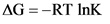Here,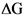is free energy change, T is temperature, R is gas constant, and K is equilibrium constant.
Because the system is thermodynamic system, we can use the above formula to calculate the equilibrium constant.
Calculate the equilibrium constant by substituting the given values.

Fundamentals
For a reaction, equilibrium constant can be calculated by using concentrations of the reactants and products.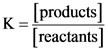Gibbs free energy is the available energy in a system. The free energy change depends on several thermodynamic parameters like enthalpy change, entropy change, and temperature.

The relationship between the equilibrium constant and Gibbs free energy change is as follows: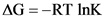Gibbs free energy change is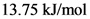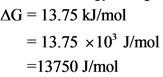Convert the free energy change from kilo joules into joules by using the following conversion factor.
1 KJ = 1000 J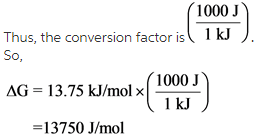Calculate the equilibrium constant by substituting the Gibbs free energy change in the formula.

Substitute the values of Gibbs energy change, temperature in the above formula.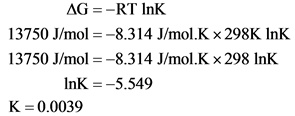Equilibrium constant (K) of the reaction is 0.0039.By substituting all the parameters in the formula, the equilibrium constant obtained with correct significant figures as 0.0039.